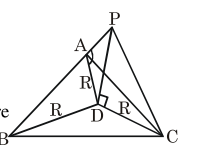# A pole stands vertically inside a triangular park ABC.

Question:

A pole stands vertically inside a triangular park ABC. Let the angle of elevation of the top of

the pole from each corner of the park be $\frac{\pi}{3}$.

If the radius of the circumcircle ot $\triangle \mathrm{ABC}$ is 2, then the height of the pole is equal to :

1. $\frac{2 \sqrt{3}}{3}$

2. $2 \sqrt{3}$

3. $\sqrt{3}$

4. $\frac{1}{\sqrt{3}}$

Correct Option: , 2

Solution:

Let $P D=h, R=2$

As angle of elevation of top of pole from

$\mathrm{A}, \mathrm{B}, \mathrm{C}$ are equal So

D must be circumcentre

of $\triangle \mathrm{ABC}$$\tan \left(\frac{\pi}{3}\right)=\frac{\mathrm{PD}}{\mathrm{R}}=\frac{\mathrm{h}}{\mathrm{R}}$

$h=R \tan \left(\frac{\pi}{3}\right)=2 \sqrt{3}$